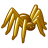# Need help using Python in a Calculate Field operation

751
2
09-08-2012 06:30 AMNew Contributor
Windows XP Pro SP3
ArcView 10.0 SP2

I have a pretty simple task that would be well suited for the attribute table's Calculate Field functionality, but I don't even know where to start as far as doing this with Python. I've written dozens of Python standalone scripts, so I don't think it's a language issue. I just don't know how to do this in the Calculate Field window.

I have five fields involved in this calculate.

Fields A1, A2, B1 and B2 are Long Integer fields. This is the logic:

if (A1 == B1 and A2 == B2):
return "A AND B IDENTICAL"
else:
return "OK"

I would like the returned value to appear in field C.

Now how in the heck would this be done in the Calculate Field window?

ALSO...is there a trove of Python code block examples somewhere?
Tags (2)
2 RepliesOccasional Contributor II
Read the help, there are many examples and tips:
http://help.arcgis.com/en/arcgisdesktop/10.0/help/index.html#/Calculate_Field_examples/00170000004s0...

In the Field Calculator check "Show Codeblock" and insert the code:
`if ([A1] == [B1] and [A2] == [B2]):    output = "A AND B IDENTICAL"else:    output = "OK"`

For "<fieldname> = " insert:
`output`Regular Contributor II

If you are going to use Python to calculate fields with lots of logic then it is much easier to use an UpdateCursor. The FieldCalculator wraps a cursor around your expression anyway so it is the same thing. I regard the FieldCalculator as a prop to use in ModelBuilder only.

The benefits of using a cursor are many:

• The logic is easier to write and understand
• It is easier to test the result and debug
• You can handle exceptions and unexpected input
• The syntax is simpler
• If you are using a join, then use a python dictionary instead for speed.
``with arcpy.da.UpdateCursor(feature_class,['A1',' B1',' A2', 'B2',' C']) as cur:      for row in cur:             if (row == row and row == row):                row = "A AND B IDENTICAL"           else:               row = "OK"         cur.updateRow(row)‍‍‍‍‍‍‍``